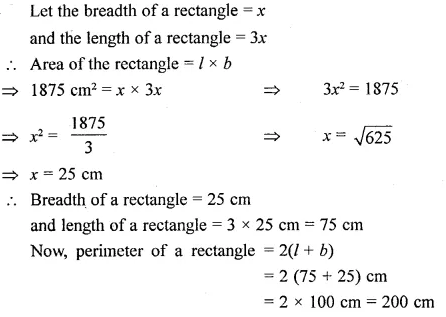# Selina Concise Mathematics Class 6 ICSE Solutions Chapter 32 Perimeter and Area of Plane Figures

## Selina Concise Mathematics Class 6 ICSE Solutions Chapter 32 Perimeter and Area of Plane Figures

Selina Publishers Concise Mathematics Class 6 ICSE Solutions Chapter 32 Perimeter and Area of Plane Figures

### Perimeter and Area of Plane Figures Exercise 32A – Selina Concise Mathematics Class 6 ICSE Solutions

Question 1.
What do you understand by a plane closed figure?
Solution: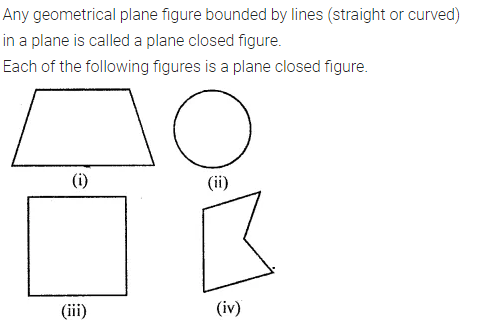Question 2.
The interior of a figure is called region of the figure. Is this statement true ?
Solution: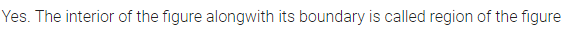Question 3.
Find the perimeter of each of the following closed figures :
Solution: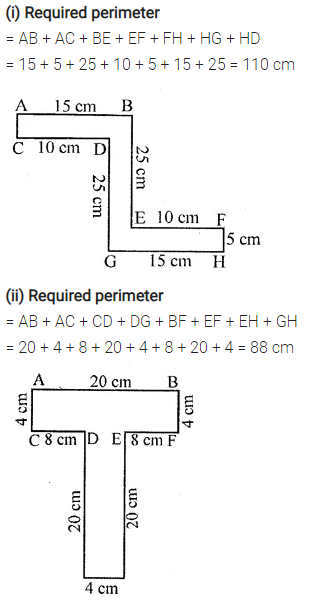Question 4.
Find the perimeter of a rectangle whose:
(i) length = 40 cm and breadth = 35 cm
(ii) length = 10 m and breadth = 8 m
(iii) length = 8 m and breadth = 80 cm
(iv) length = 3.6 m and breadth = 2.4 m
Solution: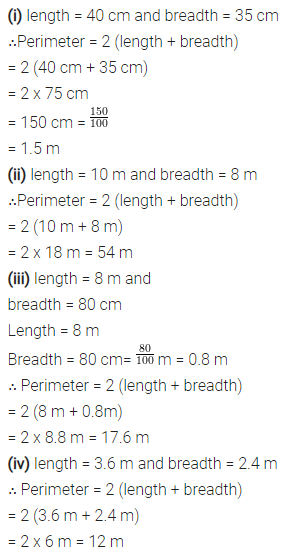Question 5.
If P denotes perimeter of a rectangle, l denotes its length and b denotes its breadth, find :
(i) l, if P = 38cm and b = 7cm
(ii) b, if P = 3.2m and l = 100 cm
(iii) P, if l = 2 m and b = 75cm
Solution:Question 6.
Find the perimeter of a square whose each side is 1.6 m.
Solution: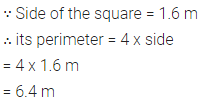Question 7.
Find the side of the square whose pe-rimeter is 5 m.
Solution:Question 8.
A square field has each side 70 m whereas a rectangular field has length = 50 m and breadth = 40 m. Which of the two fields has greater perimeter and by how much?
Solution:Question 9.
A rectangular field has length = 160m and breadth = 120 m. Find :
(i) the perimeter of the field.
(ii) the length of fence required to enclose the field.
(iii) the cost of fencing the field at the rate of ? 80 per metre.
Solution: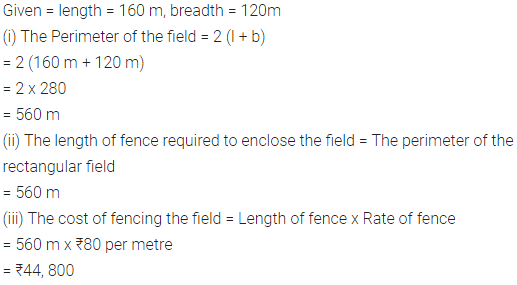Question 10.
Each side of a square plot of land is 55 m. Find the cost of fencing the plot at the rate of ₹32 per metre.
Solution:Question 11.
Each side of a square field is 70 cm. How much distance will a boy walk in order to make ?
(i) one complete round of this field?
(ii) 8 complete rounds of this field?
Solution:Question 12.
A school playground is rectangular in shape with length = 120 m and breadth = 90 m. Some school boys run along the boundary of the play-ground and make 15 complete rounds in 45 minutes. How much distance they run during this period.
Solution:Question 13.
Mohit makes 8 full rounds of a rect-angular field with length = 120 m and breadth = 75 m.
John makes 10 full rounds of a square field with each side 100 in. Find who covers larger distance and by how much?
Solution:Question 14.
The length of a rectangle is twice of its breadth. If its perimeter is 60 cm, find its length.
Solution:Question 15.
Find the perimeter of :
(i) an equilateral triangle of side 9.8 cm.
(ii) an isosceles triangle with each equal side = 13 cm and the third side = 10 cm.
(iii) a regular pentagon of side 8.2 cm.
(iv) a regular hexagon of side 6.5 cm.
Solution:Question 16.
An equilateral triangle and d square have an equal perimeter. If the side of the triangle is 9.6 cm; what is the length of the side of the square?
Solution: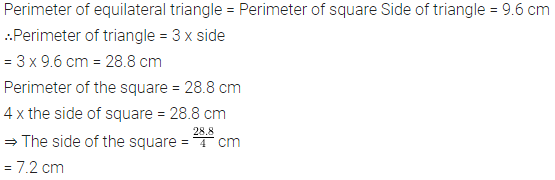Question 17.
A rectangle with length = 18 cm and breadth = 12 cm has same perimeter as that of a regular pentagon. Find the side of the pentagon.
Solution: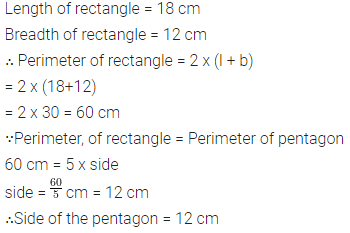Question 18.
A regular pentagon of each side 12 cm has same perimeter as that of a regular hexagon. Find the length of each side of the hexagon.
Solution:Question 19.
Each side of a square is 45 cm and a rectangle has length 50 cm. If the perimeters of both (square and rectangle) are same, find the breadth of the rectangle.
Solution: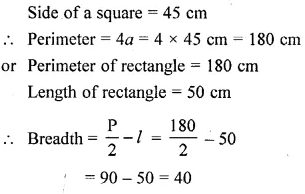Question 20.
A wire is bent in the form of an equilateral triangle of each side 20 cm. If the same wire is bent in the form of a square, find the side of the square.
Solution:### Perimeter and Area of Plane Figures Exercise 32B – Selina Concise Mathematics Class 6 ICSE Solutions

Question 1.
Find the area of a rectangle whose :
(i) length = 15 cm breadth = 6.4 cm
(ii) Length = 8.5 m breadth = 5 m
(iii) Length = 3.6 m breadth = 90 cm
(iv) Length = 24 cm breadth =180 mm
Solution: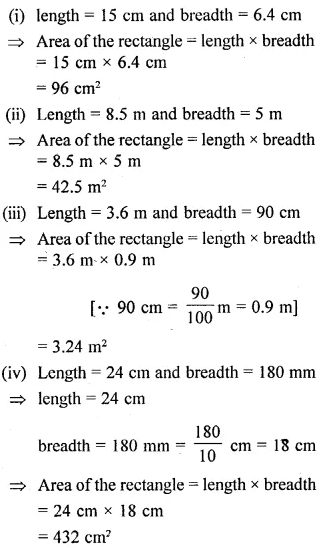Question 2.
Find the area of a square, whose each side is :
(i) 7.2 cm
(ii) 4.5 m
(iii) 4.1 cm
Solution: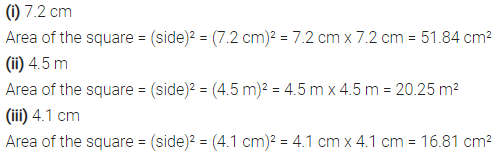>

Question 3.
If A denotes area of a rectangle, l represents its length and b represents its breadth, find :
(i) l, if A = 48 cm² and b = 6 cm
(ii) b, if A = 88 m² and l = 8m
Solution:Question 4.
Each side of a square is 3.6 cm; find its
(i) perimeter
(ii) area.
Solution:Question 5.
The perimeter of a square is 60 m, find :
(i) its each side its area
(ii) its new area obtained on increasing
(iii) each of its sides by 2 m.
Solution: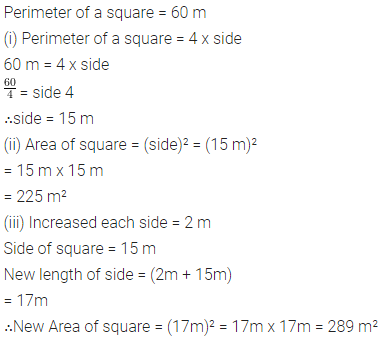Question 6.
Each side of a square is 7 m. If its each side be increased by 3 m, what will be the increase in its area.
Solution:Question 7.
The perimeter of a square field is numerically equal to its area. Find each side of the square.
Solution:Question 8.
A rectangular piece of paper has area = 24 cm² and length = 5 cm. Find its perimeter.
Solution:Question 9.
Find the perimeter of a rectangle whose area = 2600 m² and breadth = 50 m.
Solution:Question 10.
What will happen to the area of a rectangle, if its length and breadth both are trebled?
Solution:Question 11.
Length of a rectangle is 30 m and its breadth is 20 m. Find the increase in its area if its length is increased by 10 m and its breadth is doubled.
Solution:Question 12.
The side of a square field is 16 m. What will be increase in its area, if:
(i) each of its sides is increased by 4 m
(ii) each of its sides is doubled.
Solution:Question 13.
Each rectangular tile is 40 cm long and 30 cm wide. How many tiles will be required to cover the floor of a room with length = 4.8 m and breadth = 2.4 m.
Solution:Question 14.
Each side of a square tile is 60 cm. How many tiles will be required to cover the floor of a hall with length = 50 m and breadth = 36 m.
Solution:Question 15.
The perimeter of a square plot = 360 m. Find :
(i) its area.
(ii) cost of fencing its boundary at the rate of ₹ 40 per metre.
(iii) cost of levelling the plot at ₹60 per square metre.
Solution:Question 16.
The perimeter of a rectangular field is 500 m and its length = 150 m. Find:
(ii) its area.
(iii) cost of ploughing the field at the rate of ₹1.20 per square metre.
Solution: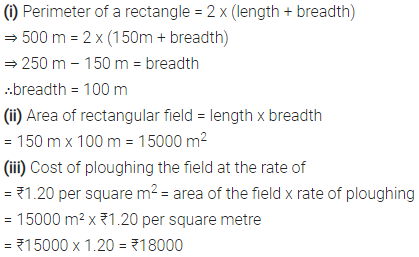Question 17.
The cost of flooring a hall of ₹64 per square metre is ₹2,048. If the breadth of the hall is 5m, find :
(i) its length.
(ii) its perimeter.
(iii) cost of fixing a border of very small width along its boundary at the rate of ₹60 per square metre.
Solution:Question 18.
The length of a rectangle is three times its breadth. If the area of the rectangle is 1875 sq. cm, find its perimeter.
Solution: